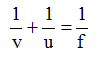# Physics

### Chapter : 3. Light Reflection

#### Important Points To Be Remember (Light Reflection)

Important Points To Be Remember
Laws of reflection :
(a) Angle of incidence is equal to the angle of reflection .
(b) The incident ray, the reflected ray and the normal all lie in the same plane.
Mirror : A smooth, highly polished reflecting surface is called a mirror. There are two types of mirrors : (a) plane mirror (b) curved mirrors
Curved mirrors are classified as spherical mirrors or parabolic mirrors depending upon the curvature of the mirror.
Concave mirror : A spherical mirror whose inner hollow surface is the reflecting surface is called concave mirror. A concave mirror forms a real, inverted image of an object if the object is placed at any place outside F. However, when the object lies between F and P, the image formed is erect and virtual.
Convex mirror : A spherical mirror whose outer bulging at surface is the reflecting surface is called convex mirror. The image formed by a convex mirror is always erect, virtual and smaller than the object whatever may be the position of the object. A convex mirror is used as a side-mirror (driver’s mirror) on automobiles.
Aperture of a mirror : The effective width of a spherical mirror from which reflection can take place is called its aperture.
Pole : The centre of a curved mirror is called its pole.
Centre of curvature : The centre of the hollow sphere of which the spherical mirror is a part is called its centre of curvature. The centre of curvature of a concave mirror is in front of it, while that of a convex mirror is behind it.
Radius of curvature : Radius of the hollow sphere of which the mirror is a part is called its radius of curvature.
Principal axis : A straight line passing through the centre of curvature and pole of a spherical mirror is called its principal axis.
Focus : A point on the principal axis of a mirror at which the rays coming from infinity meet or appear to meet is called its focus. Focus is denoted by F.
Focal length : The distance between the pole of a spherical mirror and the focus is called the focal of a spherical mirror.
Real image : The image which can be obtained on a screen is called a real image. A real image is formed only when the reflected or refracted rays actually meet at a point.
Virtual image : The image which can be seen into a mirror but cannot be obtained on a screen is called a virtual image. A virtual image is formed only when the reflected or the refracted rays appear to come from a point.
Sign convention for spherical mirrors :
According to the sign convention for mirror, the focal length of a concave mirror is negative and that of a convex mirror is positive.
Mirrors formula : The relationship,is called the mirror formula.
Magnification : The ratio of the size of the image to that of the object is called magnification. For a mirror, magnification (M) is given by,Conclusions From The Sign Convention
For spherical mirror :
Table